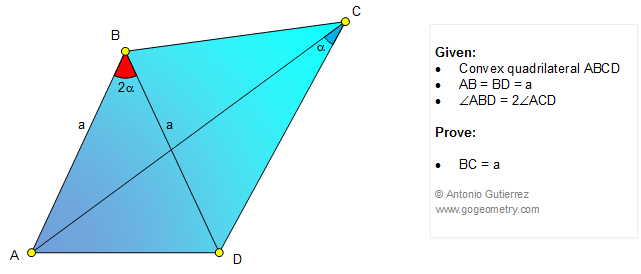Online Geometry Problem 1079: Convex Quadrilateral, Isosceles Triangle, Diagonals, Double Angle. Level: High School, SAT Prep, College, Mathematics Education

< PREVIOUS PROBLEM  |  NEXT PROBLEM >

 The figure below shows a convex quadrilateral ABCD with AB = BD and angle ABD = 2.angle ACD. Prove that BC = AB. Try to use elementary geometry (no trigonometry). See also: Problem 1079 in motionHome/a> | Search | Geometry | Problems | All Problems | Open Problems | Visual Index | 10 Problems | 1071-1080 | Triangles | Quadrilaterals | Angles | Double Angle | Email | Solution / comment | by Antonio Gutierrez Last updated: Feb 7, 2015